# Nyquist Stability Criterion: What is it? (Plus Matlab Examples)

Contents

## What is Nyquist Stability Criterion?

Nyquist stability criterion (or Nyquist criteria) is defined as a graphical technique used in control engineering for determining the stability of a dynamical system. As Nyquist stability criteria only considers the Nyquist plot of open-loop control systems, it can be applied without explicitly computing the poles and zeros of either the closed-loop or open-loop system.

As a result, Nyquist criteria can be applied to systems defined by non-rational functions (such as systems with delays). Unlike Bode plots, it can handle transfer functions with singularities in the right half-plane.

Nyquist Stability Criterion can be expressed as:

Z = N + P

Where:

• Z = number of roots of 1+G(s)H(s) in right-hand side (RHS) of s-plane (It is also called zeros of characteristics equation)
• N = number of encirclement of critical point 1+j0 in the clockwise direction
• P = number of poles of open loop transfer function (OLTF) [i.e. G(s)H(s)] in RHS of s-plane.

The above condition (i.e. Z=N+P) is valid for all the systems whether stable or unstable.

Now we will explain this criterion with examples of Nyquist stability criterion.

## Nyquist Stability Criterion Examples

### Nyquist Criterion Example 1

Consider an open-loop transfer function (OLTF) asIs it a stable system or an unstable. Perhaps most of you will say it is an unstable system because one pole is at +2. However, note that stability depends on the denominator of the closed-loop transfer function.

If any root of the denominator of the closed-loop transfer function (also called characteristics equation) is at RHS of the s-plane then the system is unstable. So in the case above, a pole at +2 will try to bring the system towards instability, but the system may be stable. Here Nyquist plot is useful to find stability.

According to Nyquist theory Z=N+P (for any system, whether it is stable or unstable).

For the stable system, Z=0, i.e. No roots of characteristics equation should be at RHS.

So for the stable system N = P.

The Nyquist plot of the above system is as shown below

#### Nyquist Plot Matlab Code

``````
s = tf('s')
G1 = 120 / ((s-2)*(s+6)*(s+8))
nyquist(G1, 'red')
``````

As per the diagram, Nyquist plot encircle the point 1+j0 (also called critical point) once in a counter clock wise direction. Therefore N= 1, In OLTF, one pole (at +2) is at RHS, hence P =1. You can see N= P, hence system is stable.

If you will find roots of characteristics equation, it will be 10.3, 0.86±j1.24. (i.e. system is stable), and Z=0. One question can be asked, if roots of characteristics equation can be found, so we can comment on the stability on that basis, then what is the need of Nyquist plot. The answer is, when software’s were not available, in those days Nyquist plot was very useful.

### Nyquist Criterion Example 2

Now take another example: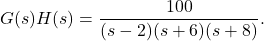Nyquist plot is as follows:

#### Nyquist Plot Matlab Code

``````
s = tf('s')
G2 = 100 / ((s-2)*(s+6)*(s+8))
nyquist(G2, 'red')
``````

From the figure, it can be found that N= 1. (Encirclement of Nyquist plot of critical point is one in counter clock wise direction)

In this example also P=1. (one pole of OLTF at RHS)

So, N=P. Hence system is stable.

(Roots of characteristics equation are 10.04, 1.72,  0.23)

### Nyquist Criterion Example 3

Now take another example: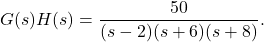Here again P=1.

Nyquist plot is as follows:

#### Nyquist Plot Matlab Code

``````
s = tf('s')
G3 = 50 / ((s-2)*(s+6)*(s+8))
nyquist(G3, 'red')
``````

You can see N=0. (No encirclement of critical point). As N is not equal to –P, hence the system is unstable. (Roots of characteristics equation are 9.32, 3.92, 1.255)  i.e. Z=1 (one pole at 1.255 is on RHS).

So, you can understand, condition Z=N+P is valid for all the systems.

### Nyquist Criterion Example 4

Now Consider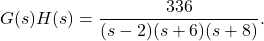If you will draw its Nyquist plot, it will pass through a critical point (-1+j0). In this case, the system is marginally stable.

You can understand ‘N’ is undefined in this case (In the present case two roots of the characteristics equation will be at the origin and one root on Left Hand Side of the s-plane. Therefore the system will be marginally stable).

In all the above examples you can see that denominator is the same, but the numerator is different, or say numerator is variable. So, let’s consider the following open loop transfer function: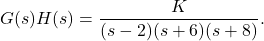If you will apply the Routh Hurwitz Criterion to characteristics equation 1+G(s)H(s), then you will find the range of ‘K’ as 96<K<336.

So, now you can understand why the systems in examples 14 are stable, unstable or marginally stable.

You can draw the Root locus of the above transfer function, it will be:

#### Nyquist Plot Matlab Code

``````
s = tf('s')
G4 = 1 / ((s-2)*(s+6)*(s+8))
rlocus(G4)
``````

Branches of Root loci is starting from 2, -6, -8, where K=0. So you can see for K<96, one pole of the closed-loop transfer function is at RHS of the s-plane, hence for K<96 system is unstable. The system is stable for 96<K<336. If you will decide K=335, then two poles of the closed-loop transfer function are complex and one pole is real; and the system will be stable.

If you will decide K=337, then two poles of the closed-loop transfer function are complex and one pole is real; but the system will be unstable. For further understanding, you can refer to articles on the root locus.

Gain margin (GM) & Phase margin (PM) are positive if the system is stable, negative if the system is unstable and both are zero if the system is marginally stable. Higher the GM & PM, more the system is stable (This is the reason, measurements of GM & PM are called relative stability).

But the above statement is true if no pole of OLTF is in RHS of s-plane. In all the above examples one pole of OLTF is at +2; in such type of systems Nyquist stability criterion is helpful.

Now we will take up few more examples:

### Nyquist Criterion Example 5

Consider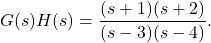Its Nyquist plot is as follows:

#### Nyquist Plot Matlab Code

``````
s = tf('s')
G5 = ((s+1)*(s+2)) / ((s-3)*(s-4))
nyquist(G5, 'red')
``````

As per the transfer function P=2 (two poles of OLTF on RHS)

As per the Nyquist plot N=0

Hence Z=N+P=2; implies that two poles of the closed loop transfer function in RHS of s-plane, hence the system is unstable.

### Nyquist Criterion Example 6

ConsiderIts Nyquist plot is as follows:

#### Nyquist Plot Matlab Code

``````
s = tf('s')
G6 = (10*(s+1)*(s+2)) / ((s-3)*(s-4))
nyquist(G6, 'red')
``````

As per the transfer function P=2 (two poles of OLTF on RHS)

As per the Nyquist plot N=2

Hence Z=N+P=0; implies that no poles of the closed loop transfer function in RHS of s-plane, hence the system is stable.

Please note that we have used the formula Z=N+P, where N=number of encirclement of critical point 1+j0 in a clockwise direction. In a few books, you may find the formula Z=N+P, where N=number of encirclement of critical point 1+j0 in a counter-clockwise direction. Both are correct.

Want To Learn Faster? 🎓
Get electrical articles delivered to your inbox every week.
No credit card required—it’s 100% free.